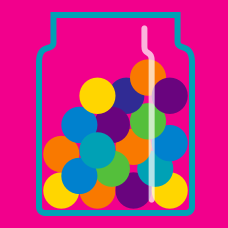Probability

# Probability - Independent events

A circle wheel labelled with integers from 1 to 10 is spun twice. If the rolls are independent, what is the probability that we will get two 6's?

Asako, Haruko, and Keiko each have a coin and are about to flip it at the same time. What is the probability that the coins of all three of the girls will land showing heads?

Dmitri, Igor, and Sergei each roll a fair standard dice only once for each. What is the probability that at least one of the three boys gets a prime number?

Asako, Haruko, and Keiko each have a coin and are about to flip it at the same time. What is the probability that exactly two of the girls have heads?

According to the weather report, there is a $50\%$ chance of rain on Saturday and a $70 \%$ chance of rain on Sunday.

If these events are independent, what is the probability that it would rain on both days?

×